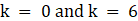SSC BOARD PAPERS IMPORTANT TOPICS COVERED FOR BOARD EXAM 2024

### Practice Set 2.5 Quadratic Equations Class 10th Mathematics Part 1 MHB Solution

Practice Set 2.5

1. Activity: Fill in the gaps and complete. (1) (2) = - 7 angle angle (3) If α, β are…
2. x^2 + 7x - 1 = 0 Find the value of discriminant.
3. 2y^2 - 5y + 10 = 0 Find the value of discriminant.
4. root 2x^2 + 4x+2 root 2 = 0 Find the value of discriminant.
5. x^2 - 4x + 4 = 0 Determine the nature of roots of the following quadratic equation.…
6. 2y^2 - 7y + 2 = 0 Determine the nature of roots of the following quadratic equation.…
7. m^2 + 2m + 9 = 0 Determine the nature of roots of the following quadratic equation.…
8. Form the quadratic equation from the roots given below. (1) 0 and 4 (2) 3 and -10 (3)…
9. Sum of the roots of a quadratic equation is double their product. Find k if equation is…
10. α, β are roots of y^2 - 2y - 7 = 0 find, (1) α^2 + β^2 (2) α^3 + β^3…
11. 3y^2 + ky + 12 = 0 The roots of each of the following quadratic equation are real and…
12. kx (x-2) + 6 = 0 The roots of each of the following quadratic equation are real and…

###### Practice Set 2.5

Question 1.

Activity: Fill in the gaps and complete.

(1)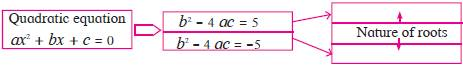(2)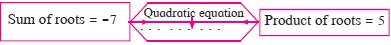(3) If α, β are roots of quadratic equation,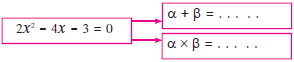(1) Roots are distinct and real when b2 - 4ac = 5, not real when b2 - 4ac = -5.

(2) x2 + 7x + 5 = 0

(3)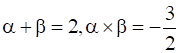Question 2.

Find the value of discriminant.

x2 + 7x – 1 = 0

⇒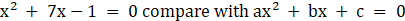⇒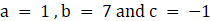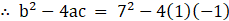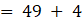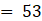Question 3.

Find the value of discriminant.

2y2 – 5y + 10 = 0

⇒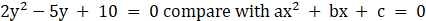⇒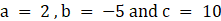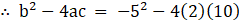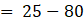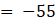Question 4.

Find the value of discriminant.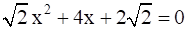⇒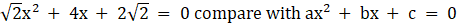⇒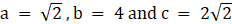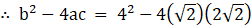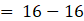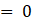Question 5.

Determine the nature of roots of the following quadratic equation.

x2 – 4x + 4 = 0

⇒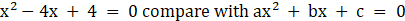⇒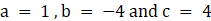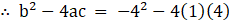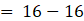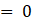∴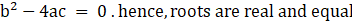Question 6.

Determine the nature of roots of the following quadratic equation.

2y2 – 7y + 2 = 0

⇒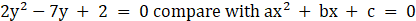⇒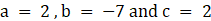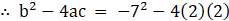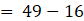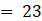∴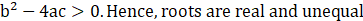Question 7.

Determine the nature of roots of the following quadratic equation.

m2 + 2m + 9 = 0

⇒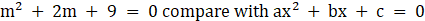⇒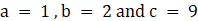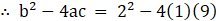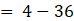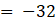∴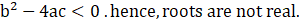Question 8.

Form the quadratic equation from the roots given below.

(1) 0 and 4

(2) 3 and -10

(3)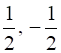(4)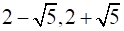(1) Let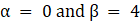∴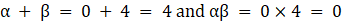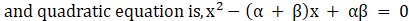∴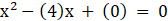∴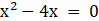(2) Let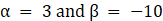∴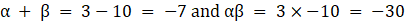∴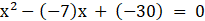∴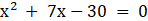(3) Let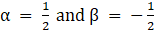∴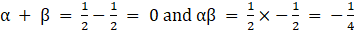∴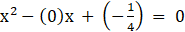∴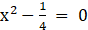∴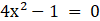(4) Let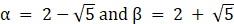∴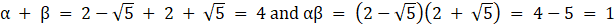∴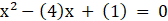∴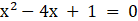Question 9.

Sum of the roots of a quadratic equation is double their product. Find k if equation is

x2 – 4kx + k + 3 = 0

According to question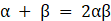⇒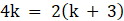⇒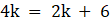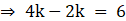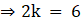⇒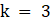Question 10.

α, β are roots of y2 – 2y – 7 = 0 find,

(1) α2 + β2

(2) α3 + β3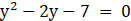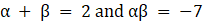(1).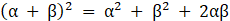⇒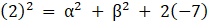⇒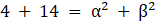⇒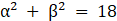(2).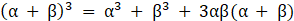⇒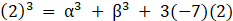⇒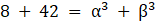⇒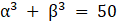Question 11.

The roots of each of the following quadratic equation are real and equal, find k.

3y2 + ky + 12 = 0

⇒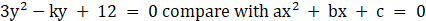⇒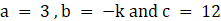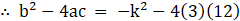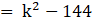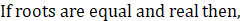∴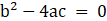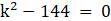⇒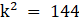⇒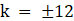∴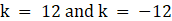Question 12.

The roots of each of the following quadratic equation are real and equal, find k.

kx (x-2) + 6 = 0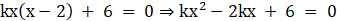⇒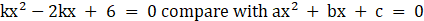⇒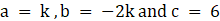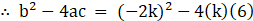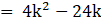∴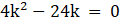⇒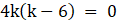⇒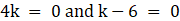∴